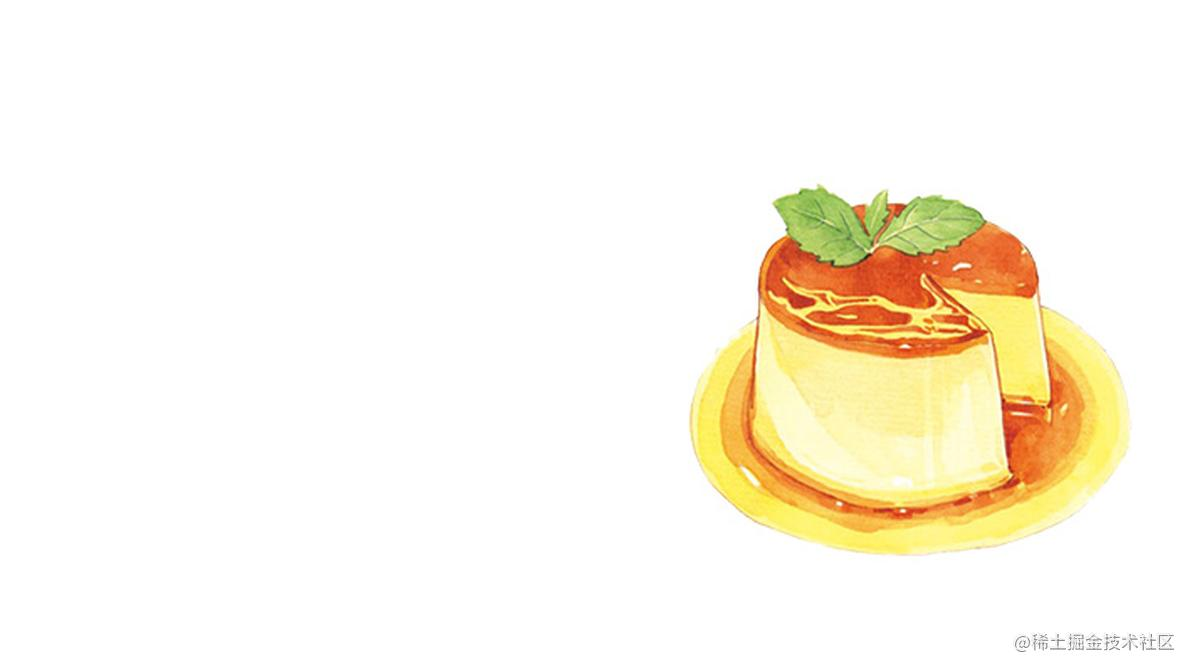# GLSL 与布丁晃动艺术# 一、位置和形状

## 2、数据传递

``````MFWobbleModel *wobbleModel = [[MFWobbleModel alloc] init];
wobbleModel.pointLT = CGPointMake(model.pointLT.x / width, 1 - (model.pointLT.y / height));
wobbleModel.pointRT = CGPointMake(model.pointRT.x / width, 1 - (model.pointRT.y / height));
wobbleModel.pointRB = CGPointMake(model.pointRB.x / width, 1 - (model.pointRB.y / height));
wobbleModel.pointLB = CGPointMake(model.pointLB.x / width, 1 - (model.pointLB.y / height));
wobbleModel.center = CGPointMake(model.center.x / width, 1 - (model.center.y / height));

1. 将当前点与中点进行连接得到一条直线，求出直线方程。
2. 求直线和贝塞尔曲线的交点。
3. 如果有交点，判读当前点是否位于交点和中心点之间，在就说明点在区域内，否则就在区域外。

``````A = y2 - y1
B = x1 - x2
C = x2 * y1 - x1 * y2

``````float getA(vec2 point1, vec2 point2) {
return point2.y - point1.y;
}

float getB(vec2 point1, vec2 point2) {
return point1.x - point2.x;
}

float getC(vec2 point1, vec2 point2) {
return point2.x * point1.y - point1.x * point2.y;
}

``````x = (1 - t)^2 * x0 + 2 * t * (1 - t) * x1 + t^2 * x2
y = (1 - t)^2 * y0 + 2 * t * (1 - t) * y1 + t^2 * y2

``````float getT1(vec2 point1, vec2 point2, vec2 point3, float a, float b, float c) {
float t;  // t = ...
return t;
}

float getT2(vec2 point1, vec2 point2, vec2 point3, float a, float b, float c) {
float t;  // t = ...
return t;
}

``````vec2 getPoint(vec2 point1, vec2 point2, vec2 point3, float t) {
vec2 point = pow(1.0 - t, 2.0) * point1 + 2.0 * t * (1.0 - t) * point2 + pow(t, 2.0) * point3;
return point;
}

``````bool isPointInside(vec2 point, vec2 point1, vec2 point2) {
vec2 tmp1 = point - point1;
vec2 tmp2 = point - point2;
return tmp1.x * tmp2.x <= 0.0 && tmp1.y * tmp2.y <= 0.0;
}

# 二、物理效果模拟

## 1、位置偏移

1. 位移只跟当前点与中心点的距离有关。距离越大，位移越小，区域边缘的位移为 0。
2. 随着与中心点距离的增加，位移呈非线形递减。

`D` 点移动到 `F` 点的时候，`E` 点会移动到 `G` 点，并且此时 `A` 点的位置不变。从俯视图来看，`D` 点的移动距离是 `HC``E` 点的移动距离是 `IJ` 。我们的最终目的就是通过 `HC` 来求 `IJ`

``````AF = acos(HC / AC) * AC
AE = acos(JC / AC) * AC
AD = (PI / 2) * AC
AG = AE * AF / AD
IJ = AC * (cos(AG / AC) - cos(AE / AC))

``````float centerOffsetAngle = acos(maxCenterDistance / maxDistance);
float currentAngle = acos(distanceToCenter / maxDistance);
float currentOffsetAngle = currentAngle * centerOffsetAngle / (PI / 2.0);
float currentOffset = maxDistance * (cos(currentOffsetAngle) - cos(currentAngle));

## 3、振幅衰减

``````model.amplitude *= 0.7;
model.amplitude = model.amplitude < 0.1 ? 0 : model.amplitude;

# 三、输入事件处理

## 1、触摸事件

``````- (void)touchesBegan:(NSSet<UITouch *> *)touches withEvent:(UIEvent *)event {
[super touchesBegan:touches withEvent:event];

CGPoint currentPoint = [[touches anyObject] locationInView:self];
currentPoint = CGPointMake(currentPoint.x / self.bounds.size.width, 1 - (currentPoint.y / self.bounds.size.height)); // 归一化
for (MFWobbleModel *model in self.wobbleModels) {
if ([model containsPoint:currentPoint]) {
self.currentTouchModel = model;
self.startPoint = currentPoint;
break;
}
}
}

- (void)touchesMoved:(NSSet<UITouch *> *)touches withEvent:(UIEvent *)event {
[super touchesMoved:touches withEvent:event];

if (self.currentTouchModel) {
CGPoint currentPoint = [[touches anyObject] locationInView:self];
currentPoint = CGPointMake(currentPoint.x / self.bounds.size.width, 1 - (currentPoint.y / self.bounds.size.height)); // 归一化
CGFloat distance = sqrt(pow(self.startPoint.x - currentPoint.x, 2.0) + pow(self.startPoint.y - currentPoint.y, 2.0));
CGPoint direction = CGPointMake((currentPoint.x - self.startPoint.x) / distance, ((currentPoint.y - self.startPoint.y) / distance));
[self startAnimationWithModel:self.currentTouchModel direction:direction amplitude:1.0];

self.currentTouchModel = nil;
}
}

## 2、加速计

``````self.motionManager.accelerometerUpdateInterval = 0.1;  // 0.1 秒检测一次
__weak typeof(self) weakSelf = self;
[self.motionManager startAccelerometerUpdatesToQueue:[[NSOperationQueue alloc] init] withHandler:^(CMAccelerometerData *accelerometerData, NSError *error) {
CMAcceleration acceleration = accelerometerData.acceleration;
CGFloat sensitivity = sqrt(pow(acceleration.x, 2.0) + pow(acceleration.y, 2.0));
if (sensitivity > 1.0) {
CGPoint direction = CGPointMake(acceleration.x / sensitivity, acceleration.y / sensitivity);
for (MFWobbleModel *model in weakSelf.wobbleModels) {
// 当前的振幅小于某个阈值才会受影响
if (model.amplitude < 0.3) {
[weakSelf startAnimationWithModel:model direction:direction amplitude:1.0];
}
}
}
}];